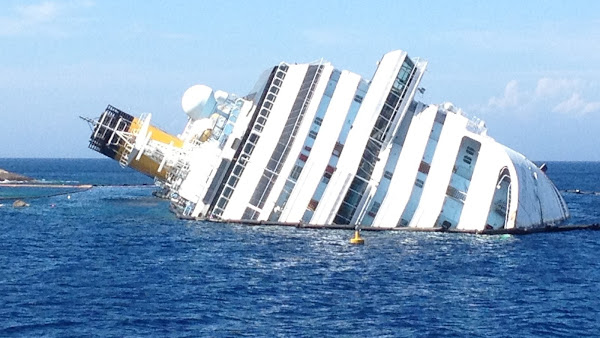## 20141111

### Physics quiz question: Costa Concordia ledge water pressure

Physics 205A Quiz 5, fall semester 2014
Cuesta College, San Luis Obispo, CA

Cf. Giambattista/Richardson/Richardson, Physics, 2/e, Problem 9.13"Costa Concordia 5"
paolodefalco75
commons.wikimedia.org/wiki/File:Costa_Concordia_5.jpg

In January 13, 2012 the cruise ship Costa Concordia grounded and partially capsized, resting half-submerged on an underwater ledge, with 32 lives lost.[*] The ledge that the Costa Concordia rested on was 20 m below sea level. (Approximate the density of sea water as ρwater = 1.0 ×103 kg/m3, and Patm = 101.3 kPa.) The water pressure at that depth is:
(A) 2.0×103 Pa.
(B) 9×104 Pa.
(C) 2.0×105 Pa.
(D) 3.0×105 Pa.

[*] wiki.pe/Costa_Concordia_disaster.

Correct answer (highlight to unhide): (D)

For static fluids, the energy density relation between pressure and changes in elevation is given by:

0 = ∆P + ρ·g·∆y,

0 = (PledgePsea level) + ρ·g·(yledgeysea level),

where the water pressure at sea level is 101.3 kPa = 1.013×105 Pa, such that:

Pledge = Psea level – ρ·g·(y ledge ysea level),

P ledge = 1.013×105 Pa – (1.0 ×103 kg/m3)·(9.80 m/s2)·(– 20 m – 0 m),

Pledge = 1.013×105 Pa + 1.96×105 Pa = 2.97×105 Pa,

which is 3.0 ×105 Pa, to two significant figures.

(Response (C) is ρ·g·∆y, which is the difference in pressure between sea level and the underwater ledge depth; response (B) is Psea level – ρ·g·∆y; response (A) is Psea level·∆y/ρ.)

Sections 70854, 70855, 73320
Exam code: quiz05mRp4
(A) : 18 students
(B) : 5 students
(C) : 33 students
(D) : 7 students

Success level: 11%
Discrimination index (Aubrecht & Aubrecht, 1983): 0.37